[BACK]
 Intelligent Automation & Soft ComputingDOI:10.32604/iasc.2023.028022Article

WOA-DNN for Intelligent Intrusion Detection and Classification in MANET Services

1Department of Computer Science and Engineering, Noorul Islam Centre for Higher Education, Nagercoil, 629180, India
2Department of Information Technology, Associate Professor, Noorul Islam Centre for Higher Education, Nagercoil, 629180, India
*Corresponding Author: C. Edwin Singh. Email: cedwinsingh22@gmail.com
Received: 31 January 2022; Accepted: 09 March 2022

Abstract: Mobile ad-hoc networks (MANET) are garnering a lot of attention because of their potential to provide low-cost solutions to real-world communications. MANETs are more vulnerable to security threats. Changes in nodes, bandwidth limits, and centralized control and management are some of the characteristics. IDS (Intrusion Detection System) are the aid for detection, determination, and identification of illegal system activity such as use, copying, modification, and destruction of data. To address the identified issues, academics have begun to concentrate on building IDS-based machine learning algorithms. Deep learning is a type of machine learning that can produce exceptional outcomes. This study proposes that WOA-DNN be used to detect and classify incursions in MANET (Whale Optimized Deep Neural Network Model) WOA (Whale Optimization Algorithm) and DNN (Deep Neural Network) are used to optimize the preprocessed data to construct a system for classifying and predicting unanticipated cyber-attacks that are both effective and efficient. As a result, secure data transport to other nodes is provided, preventing intruder attacks. The invaders are found using the (Machine Learning) ML-IDS and WOA-DNN methods. The data is reduced in dimensionality using Principal Component Analysis (PCA), which improves the accuracy of the outputs. A classifier is used in forward propagation to predict whether a result is normal or malicious. To compare the traditional and proposed models’ effectiveness, the accuracy of classification, detection of the attack rate, precision rate, and F-Measure, Recall are utilized. The proposed WOA-DNN model has higher assessment metrics and a 99.1% accuracy rate. WOA-DNN also has a greater assault detection rate than others, resulting in fewer false alarms. The classification accuracy of the proposed WOA-DNN model is 99.1%.

Keywords: Intrusion detection system; whale optimization algorithm; deep neural network; mobile ad-hoc networks; forward and back propagation

1  Introduction

Based on where the IDS is deployed, it is classified as Host-based IDS (HIDS), Network-based IDS (NIDS), Intrusion solutions include the Virtual Machine Monitor/Hypervisor-based IDS (VMM-IDS), as well as Collaboration IDS. To identify intrusions inside the VM or Host, HIDS is implemented . The HIDS analyses log files and audit the system’s extracted operation to improve intrusion detection. NIDS is placed at network entry points to identify system and system behavior irregularities. By studying network protocols and traffic, NIDS detects intrusions. In the existing technology, matching algorithms are employed for analysis. The matching features are generally strings, port As a result, characteristics such as packet data header attributes are used, and it is one of the most effective strategies for increasing the NIDS’s consistency and punctuality. Particle swarm is the current feature selection method. n genetic algorithm, gray wolf algorithm, cuckoo algorithm, etc. VMM-IDS observes the functioning of VMs from outside via VMM/hypervisors.

Machine learning is being used to build IDSs, according to scientists approaches to address the aforementioned concerns. Machine learning is an artificial intelligence technique for extracting useful information from enormous datasets automatically. Machine learning-based IDSs can reach suitable research was motivated when enough training evidence is accessible, and machine learning models have the knowledge to recognize attack variations and distinct threats . When working with enormous amounts of data, deep learning approaches outperform typical machine learning techniques. On the other hand, supervised neural algorithms, may automatically train feature representations from raw data and then output results; they are end-to-end and practical. The deep structure, which has numerous hidden layers, and others. Deep learning is a more advanced way for extracting features, learning, and perceiving machines. The depth and architecture of the human brain-inspired deep learning. Deep learning has demonstrated excellent outcomes in AI functions, such as network congestion prediction, intrusion detection, data flow analysis, and malware categorization. Deep learning outperforms previous IDS approaches in certain domains where patterns are getting increasingly unstructured and heterogeneous. This paper proposes Intrusion detection and classification system MANET using WOA -DNN Model.

The following is how the rest of the paper is organized: Section 2 discusses related IDS research that use deep learning, section 3 describes the proposed model, section 4 illustrates the proposed model’s experiment results, and section 5 wraps up the paper.

2  Related Work

There are numerous study areas for intrusion detection systems that use deep learning and machine learning approaches; some of these relevant works are listed below:

The paper  based on the DNN algorithm, (K. Amarasinghe et al., 2018) suggested an intrusion detection system that detects attacks. (M. Maithem et al., 2021) makes use of the KDD CUP 99 dataset. The dataset is first preprocessed to remove text values, which the DNN algorithm cannot analyze . (W. F. Zheng 2020) The DNN receives the preprocessed data and employs forward and backward propagation. For multi-class categorization, the experimental investigation demonstrates 99.98 percent accuracy.  To fight attacks, the KDD Cup 99 database is utilised to evaluate a DNN-based abnormality detection system. The activation function of the hidden layers was ReLU, which was utilised to form a neural net with four hidden units and Adam optimizer for Backpropagation. The accuracy of the model was 99.08%. (M. S. E. Sayed et al., 2021) the proposed model in  used an Deep convolution network-based intrusion prevention system (CNN). The dataset KDD Cup 99 was utilised and two dimensionalities were performed on the dataset and showed a 97.7% detection rate.  This paper (S. Rajabi, et al., 2020) proposed a CNN-based intrusion detection algorithm with two convolution layers and pooling layers. To improve the network speed a batch normalization layer is included after each convolution layer. To train the model SGD and Adam optimizers were used and the average precision is 0. 9507. (Z. Wang et al., 2021) proposed approach  was based on the firefly algorithm for feature selection and fast learning networks. Features selected using firefly algorithms are used as inputs for FLN (Fast Learning Network) which detects the network intrusions. The neural network includes three layers. For evaluating the accuracy of the model, a confusion matrix was used. This model has an accuracy of 99.9%.  This work (A. Thirumalairaj et al., 2020) proposed a deep intrusion detection that is incorporated using SDAE-ELM for NIDS and DBN-Softmax For HIDS, there is a model. The (Stacked Denoising Autoencoder-Extreme learning machine) SDAE-ELM model reduces noise in NIDS datasets while also increasing speed. To improve the model’s computational performance, the SDAE-ELM and DBN-Softmax were trained utilising the Mini-Batch gradient technique capacity. When compared to conventional machine learning, this one outperforms them, the (Z. Ye et al., 2019) proposed models have shown better outcomes but the drawback in SDAE-ELM seems to be detecting intrusions in small datasets was poor. The drawback of DBN-SoftMax, it takes a long time for training large datasets . (S. Mirjaliliet al., 2016) The proposed HCSTS-DNN (Hybrid Cuckoo Search Optimization based Tuning Scheme for Deep Neural Network) model is based on a hybrid cuckoo search algorithm that is integrated with L-BFGS and which is used to optimize the DNN parameters. The test dataset is introduced to the DNN structure after the model has been trained to detect any intrusions. It has a 99.95 percent accuracy for NSL-KDD 2015 datasets and 99.98 percent accuracy for CICIDS 2017 datasets. . (W. Sun et al., 2021) suggested a three-branch anchoring network with part-awareness and parallel learning On the VehicleID and VeRi-776 datasets, the TBE-Net beats state-of-the-art approaches in extensive testing. . (W. Sun et al., 2021) proposed a new YOLOv3-based real-time small object recognition (RSOD) system that improves small object detection performance by I employing feature maps from a relatively shallow layer with more perfectly alright data for definitely intended (ii) enhancing the sound waves layer in the Squeeze-and-Excitation long short - term memory to adjust the feature responses of each channel more precisely; (iii) assigning weights to FPN output features .

This paper proposed a grasshopper optimization algorithm to optimize the precision of finding intrusions using SVM.GOA is used to find the optimal parameters of SVM to increase classification accuracy. The fitness function is the IDS accuracy of SVM. It used KDD Cup 99 dataset. GOA-SVM has an accuracy of 97.7%.

3  Proposed Model

The Proposed WOA-DNN in the MANET method involves the following modules as shown in Fig. 1 as data preprocessing, optimization, and Detection and classification.Figure 1: Whale optimized deep neural network model (WOA-DNN)

3.1 Data Preprocessing Module

Deep Learning algorithms work with numerical values, the data for the study is One-hot encoding was used to transform the data to quantitative numbers and it turns categorical data into numerical values. Data normalization and other processes are included in the data preparation module to ensure that datasets meet input data requirements. Principal Component Analysis (PCA) is used to normalize data. PCA decreases the data’s dimensionality, improving the accuracy of the outputs.

3.1.1 One Hot Encoding

One Hot encoder is used for converting the text attributes to numerical values. Numerical values must be entered in the input layer of the DNN. Numerical values range from 0 to 1. Encoding is used to convert data so that it is easy for the computer to understand. The label values are translated into 0 or 1 and the text property is converted into a new column. E.g., the protocol type attribute column in KDD Cup 2017 dataset is shown in Tab. 1.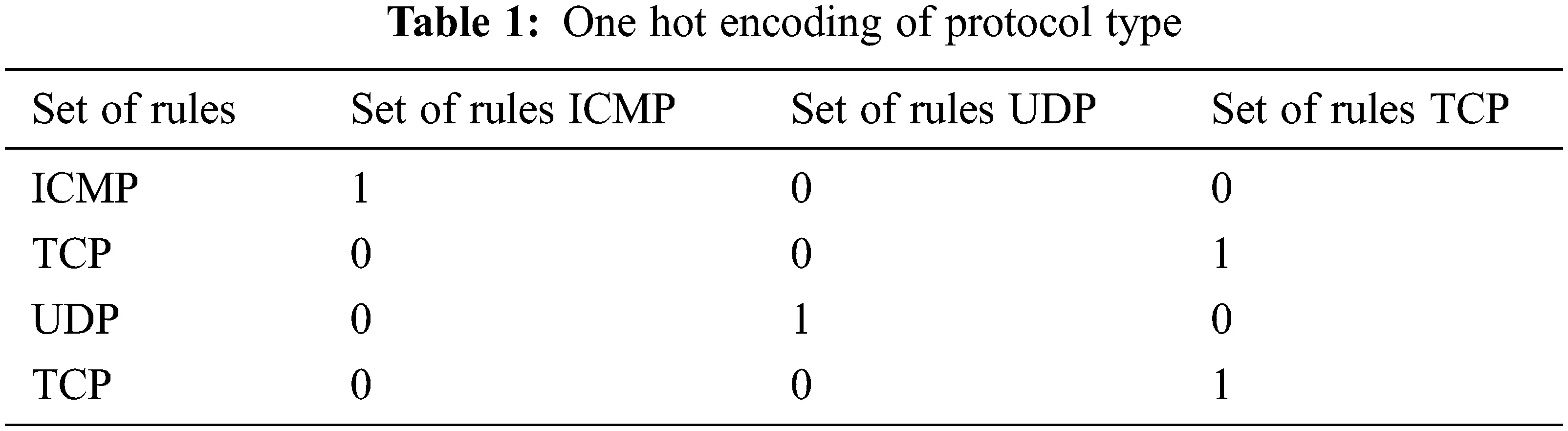3.1.2 Data Normalization

The training and test data are trained to reduce the dimensional impact of each dataset. The value in the dataset is normalized in the range 0 to 1. It is used to eliminate the negative effect of data with higher values since it affects the accuracy of the classification model. PCA is mainly used in preprocessing and data analysis. PCA involves the following steps.

•   Select the initial dataset Y. Standardize the raw input data with mean = 0 and variance = 1. Each dataset has n objects and each object has m variables

Yij=aijaj¯bj, i=1,2,nandj=1,2,m (1)

where a¯j=i=1naijn , aj2=i=1n(aijaj¯)2n1

•   Compute the covariance matrix of dimensions.

C=YTYn1 (2)

•   Obtain the Eigenvectors and eigenvalues e1≥e2…. ≥em of C from the covariance matrix.

e1=[α11α21αm1],e2=[α12α22αm2],em=[α1mα2mαmm] (3)

•   The first k eigenvectors that correspond to the k biggest Eigenvalues are chosen after the Eigenvalues are sorted in descending order to form a matrix with dimension j × k.

•   The matrix of projection W is constructed from the specified eigenvectors.

•   The dataset is transformed through W to obtain the new k-dimensional subspace.

Pi=α1iY1+α2iY2+.+αniYmwherei=1,..m (4)

PCA reduces the initial data dimension while keeping as much variance in these samples as possible by mapping high-dimensional data to low-dimensional space and applying the methods below.

3.2 Optimization Module

The dataset from the preprocessing module is then optimized using a whale optimization algorithm (WOA). The data is optimized by removing irrelevant dimensions thereby reducing the execution time and also performing numerical optimization on the preprocessed data.

3.2.1 Whale Optimization Algorithm

The whale optimization algorithm (WOA)  is a natural-inspired meta-heuristic method for solving problems. In their search process, most meta-heuristics have a similar trait. The procedure is divided into two parts: exploration and commercialization. It is based on the hunting behaviour of humpback whales. ‘Humpback whales’ are a type of whale that lives in the unusual hunting style is the most fascinating feature of their biology. The bubble-net feeding technique is a type of foraging activity. At the water surface, humpbacks like to eat krill or small fish. This foraging has been observed to be done by forming distinct bubbles in a circle. This hunting approach is usually connected with two maneuvers. The first is called ‘upward-spirals,’ in which the whale dives 12 meters below the surface and swims towards the surface, forming spiral-shaped bubbles; the second consists of three stages: lobtail, catch loop, and coral loop, and is more sophisticated. This one-of-a-kind spiral bubble-net hunting activity is only visible in humpback whales.

Investigation and utilization are the two phases of WOA. Exploring is focused with a broad hunt for the perfect solutions, whereas exploitation is focused with a narrow search for the greatest solutions. concerned with a more focused local search. Exploitation involves searching a region of control with the search space to enhance the solution. While in exploration, it searches a considerably larger area of the search space in the hopes of finding other promising solutions that have yet to be developed. WOA uses an optimization strategy to find the best solution, comparable to prey hunting and positioning the prey in a certain location. The WOA starts with a population of randomly created whales (solutions) in various locations. At start, the search agents change their locations depending on a search agent chosen at random. After the first round, the search agents adjust their placement based on the best response found. If the value of |A| is more than one, a random search agent is chosen to aid in research. |A| is set to |A| 1 when the best solution is found. This leads to manipulation, which makes WOA a good planner when contrasted to all the search agents converging. Probing for prey, bubble-net hunting, and enveloping the prey are the three phases of whale hunting.

Surrounding the Prey: Whales alter their location based on the optimal search agent, duplicating the encircling behavior during optimization. The encircling is mathematically modeled by the following equations:

D=|C.X(t)X(t)| (5)

where t represents the current iteration, A and C represent coefficient matrices, X represents the coordinates, and X represents the optimal solution’s position vector, which is changed if a better solution can be found. The coefficient vectors A and C are calculated using Eqs. (7) and (8), accordingly (8).

A=2a.ra (7)

C=2.r (8)

where (r) is a vector space that ranges between [0,1] and vector () declines linearly from 2 to 0 for rounds.

Bubble Net Hunting: Whale attack behavior is influenced by the bubble net attack tactic. Bubble net hunting of whales is shown in Fig. 2. This strategy considers two primary approaches: the shrinking position update method that encircles and spirals. The whales use both methods at the same time to swim around their prey. As a result, it is estimated that there is a 50% chance of choosing between the two to update their location during optimization. The mathematical model is as follows:

X(t+1)=X(t)A.Difp<0.5 (9)

D,.ebl.cos(2πl)+X(t)ifp0.5 (10)Figure 2: Bubble net hunting of whales

Probing for Prey (Investigation Phase): During the discovery phase, the search agents can change the vector are activated to look for better solutions. A. |A| ≥1 as a result, the The search agent makes a considerable departure from the search space. Instead of employing the best search agent, search agents use a randomly selected search agent to update their locations in the define stage. The equation can be used to model the search method. Fig. 3 depicts the whale optimization method’s pseudocode.

D=|CXrandX| (11)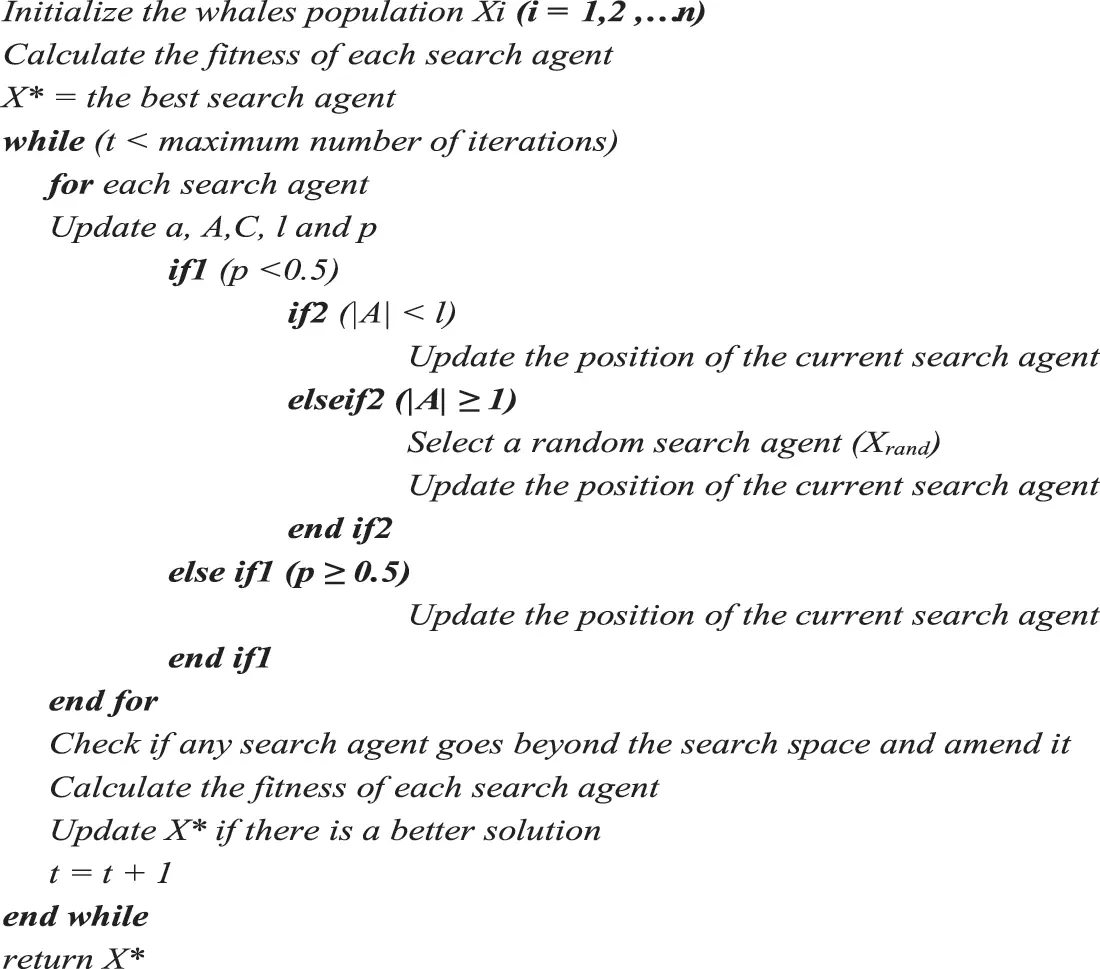Figure 3: Pseudocode for WOA algorithm

3.3 Detection and Classification Module

DNN (Deep Neural Network) is applied to the data from the optimization module for the classification process DNN is used since it can handle big data. DNN has input, hidden, and output layers as depicted in Fig. 4. The ADAM optimizer was utilised as the model’s optimization technique.

DNN examines a variety of patterns to provide the best possible results. Auto-encoders are used to represent hierarchical structures in DNN. DNN is made out of several Nodes are arranged in layers. Each level in the networks is completely linked to the layer above it. A fully connected Deep A neural network is comprised of an input layer, hidden layers, and output units . One input layer, three hidden layers, and one output layer make up the CIDCS Deep Convolutional Neural Network.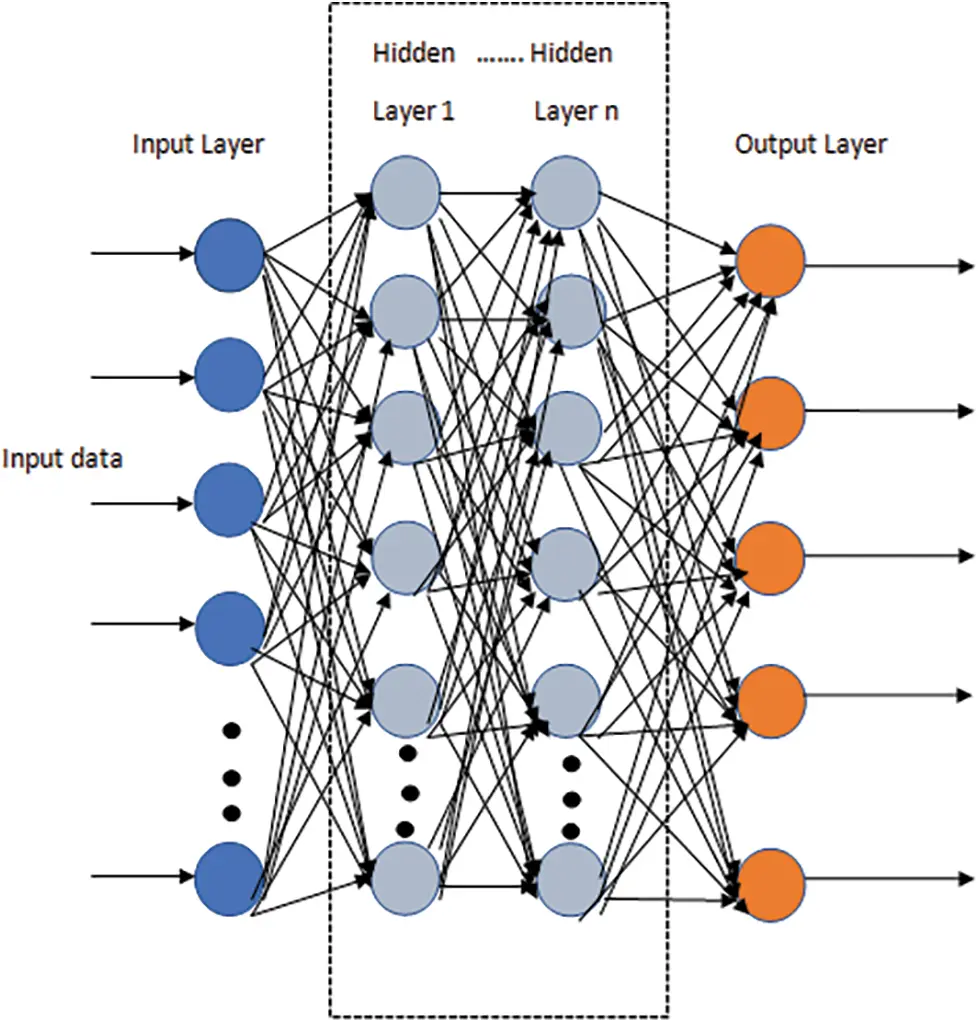Figure 4: DNN structure

3.3.1 DNN Structure

Input Layer: It prepares the information for DNN. Several hidden layers convey the instances from the input layer, as well as weight and bias, to the neurons. In the NSL-KDD dataset, 41 nodes in the input layer represent the number of input characteristics.

Hidden Layer: It’s the layer that sits between the input and output layers and is responsible for all computations. Many activation functions, such as Relu, Sigmoid, and others, are used to activate the hidden layers. In the buried layers, the ReLU activation function is applied.

The categorization results are displayed in the output layer. The circumstance is described using the adjectives “regular” and “attack.” The set of target subclasses has a direct relationship with the output layer. Only one neuron is coupled to the output units if the classification model is binary. Eventually, the connections will be disrupted. are chosen based on the multi-class problem. The Softmax is used for multiclass classification, whereas the sigmoid function is used for classification algorithm.

3.3.2 Forward Propagation

Forward propagation uses a classifier to predict results that are either normal or attack. Then an activation function is used, these results will be given as input to the function. The study of how a neuron operates inside the human brain led to the hypothesis of an activation function, in which the neuron gets active above a particular level described as the activation potential. This also limits the variety of possible outcomes. The most commonly used activation functions are Sigmoid, ReLU, and softmax. In the buried layers, the Linear transfer function is provided as.

f(y)=0fory0 (13)

f(y)=xfory>0 (14)

The output layer’s Softmax function is described as follows:

σ(z)j=ezjk=1kezkforj=1,2,3,..k (15)

where,

Z- The soft - max stored procedure input vector is built up of (z0, … zK)

Zj- All of the zi values are members of the soft - max stored procedure input vector, and they can be any real number, positive, zero, or negative. For example, a neural network might produce a vector like (–0.62, 8.12, 2.53), which isn’t a legitimate probability, necessitating the use of the fully connected layers.

ezj - Each member of the input vector is subjected to the conventional exponential curve. This generates a positive value greater than zero, which will be very little if the input is low and very huge if the input is large. However, it is not fixed in the range (0, 1), which is what a likelihood must be.

k=1kezk - The normalization term is the term at the bottom of the equation. This verifies that all of the function’s target value add up to 1 and are all in the range (0, 1), resulting in a valid probability distribution.

In a multi-class classification, k is the set of classes.

3.3.3 Back Propagation

Backpropagation is used to train a DNN by Modifying weights and bias. BP backpropagates the error from the back to the front and uses it to adjust the weight that has an impact on the output. At each step of the backdrop, the weights at each layer are updated. It includes loss function and Optimizers. The loss function decreases the value to obtain the optimal values for the model parameters. It has many parameters for every model and the configuration of the model is characterized by the parameter values which are denoted as bias and weight in the NN. The model is estimated by using the loss function. To achieve the optimal value for each parameter, the loss function must be optimized. The loss function must reach the optimal value of the parameter of the model (weight and bias). To get the best parameter value optimizer is used. The most common Loss Functions are RMS prop, Batch gradient descent, Adam and Stochastic gradient descent. The ADAM optimizer was utilised as the model’s optimization algorithm. When using the spine method to learn the model, the learning epoch is set to 1000 (Epoch is the number of times the information is transmitted through the perfect back method core network for retraining) and the batch size is set to 1 million. The methodology employs a categorization paradigm, with the primary purpose of classifying each packets into one of two categories: normal or assault.

4  Experiment & Analysis

The results from the WOA-DNN model are simulated using two datasets NSL-KDD.

4.1 Dataset Used

The first dataset has 41 features. Intruder behavior has 4 behaviors which are Denial of Service (DoS), unauthorized access to superuser privileges by unprivileged users (U2R), probing, and unauthorized access from remote to the local system (R2L). The target class has 5 values for attack DOS, probe, R2L, U2R, and normal. If the value for the target class is something other than 0, then there is an intrusion show in Tab. 2.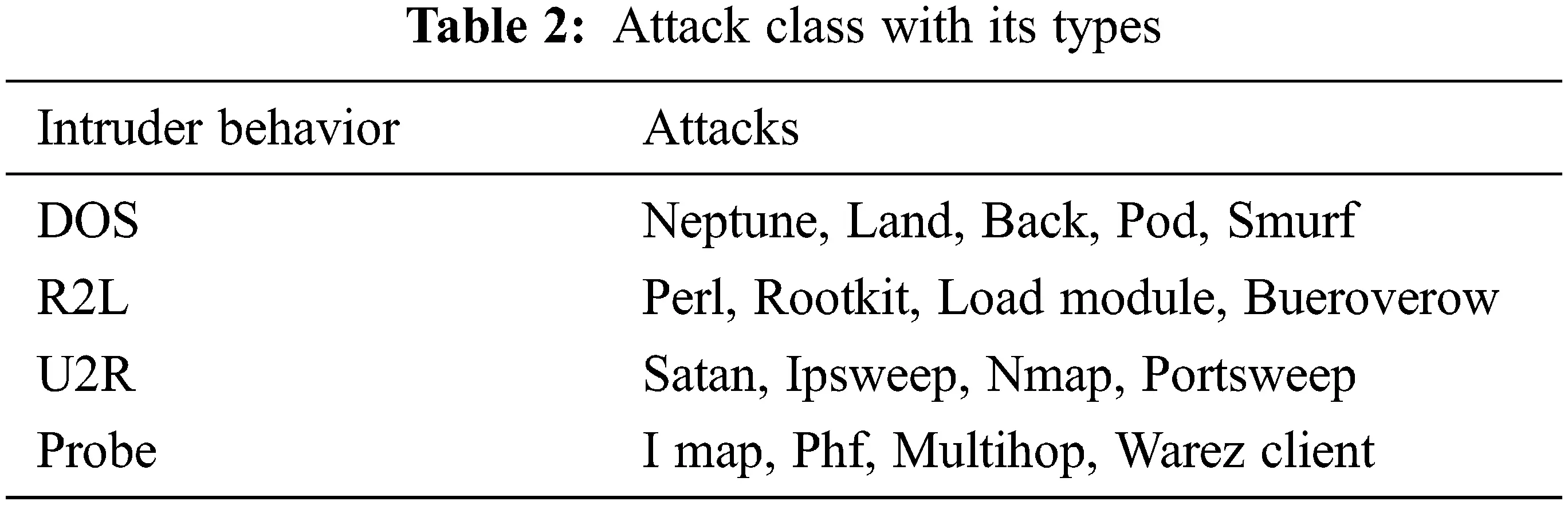The dataset is preprocessed to convert text attributes to numerical values for increasing the accuracy of the classification. One-hot encoding is used to achieve this. Then PCA is applied to achieve data dimensionality reduction and the next optimization is done using the WOA algorithm. After that, the DNN The enhanced data is classified using a classifier. To evaluate the results of the conventional and suggested models, the efficiency of classification, detection of the attack rate, precision rate, and F-Measure are utilised show in Fig. 5.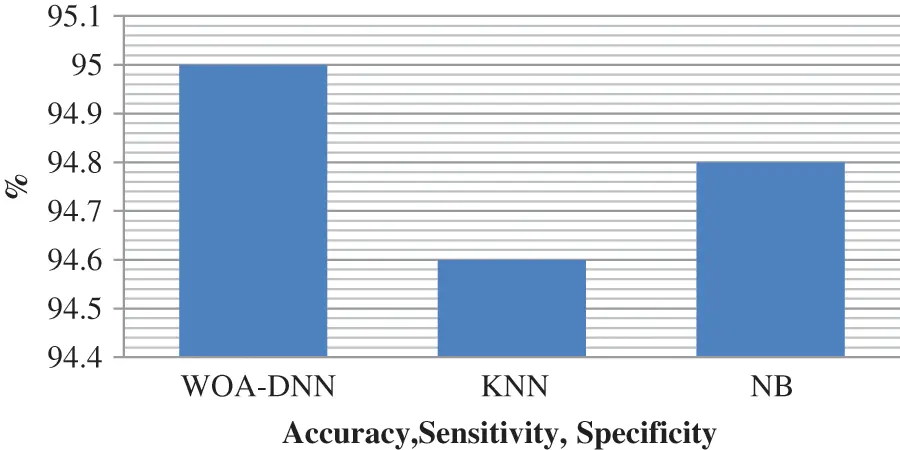Figure 5: Evaluation of DNN classifier with ML classifier

4.2 Evaluation Metrics

True Positive (TP): Abnormal connections which are classified as intrusive. Normal interconnections that are falsely positive (FP) are considered invasive. True Negative (TN): Connectors that are recognized as regular are categorized as such. False Negative (FN): abnormal connections are mistaken for good interactions.

•   Detection of Attack Rate (AR): It denotes the number of attacks detected to the number of attacks that existed.

AR=TPTP+FN (16)

•   Accuracy of the classification (AC): It denotes instances that are classified correctly to the total existing number of instances. It is used to check whether the system generates correct alarms and not false alarms.

AC=TP+TNTP+FP+TN+FN (17)

•   Precision Rate (PR): It indicates the positive values that are truly positive. A higher value shows less FPR.

PR=TPTP+FP (18)

•   F-Measure: It analyzes the accuracy of the proposed WOA-DNN system based on recall and precision rates.

FMeasure=2TP2TP+FP+FN (19)

The training time taken by the various models is depicted in Fig. 6. When compared to previous models, our suggested approach requires less training time show in Tab. 3.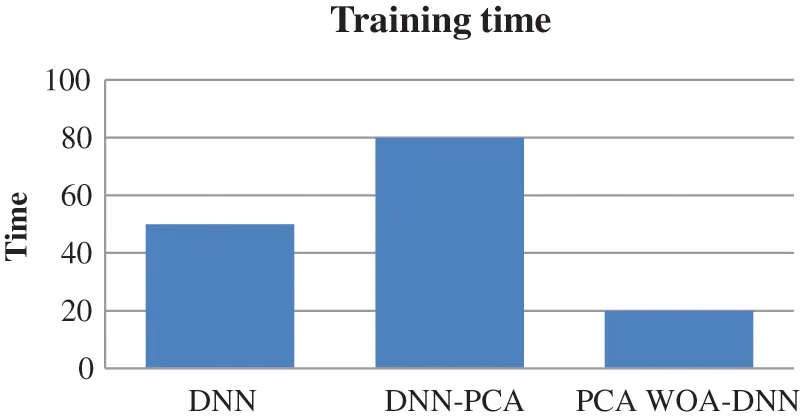Figure 6: Training time of models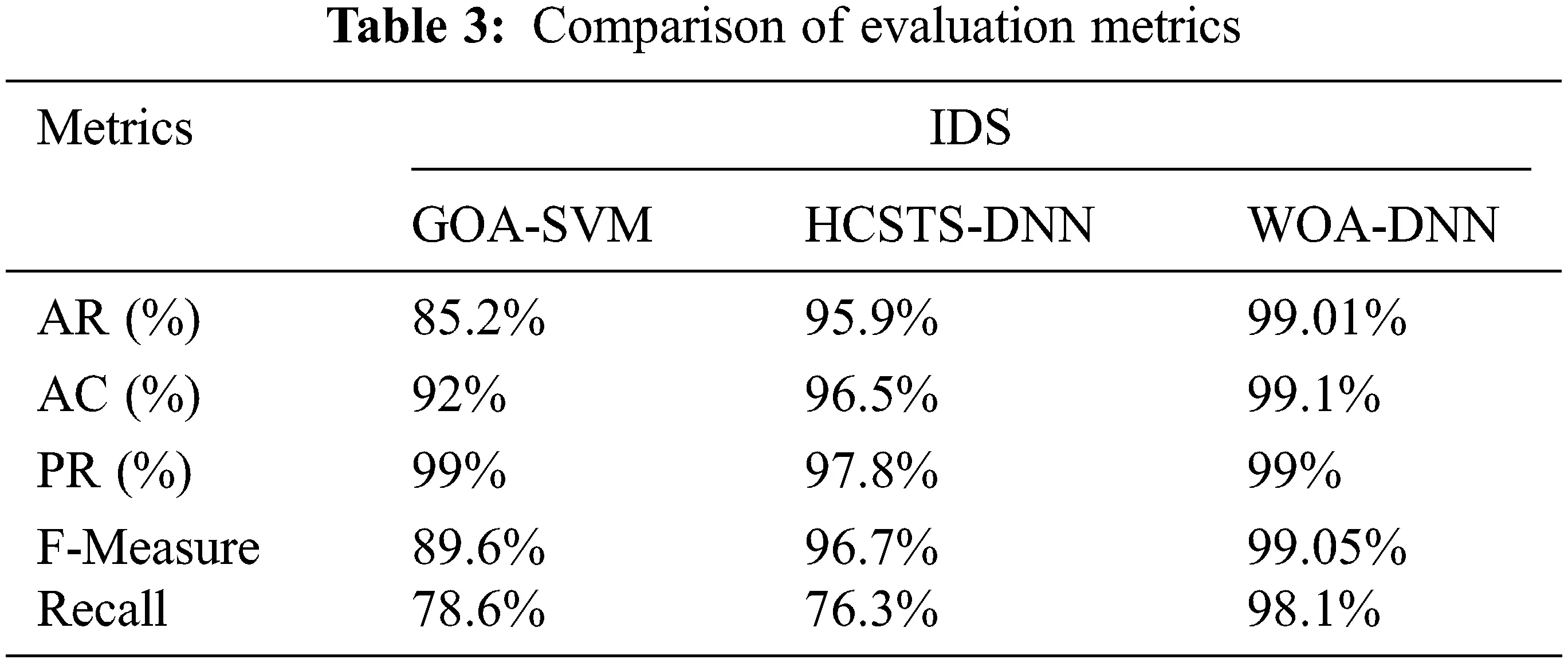Using the testing and training datasets, Fig. 7 depicts the accuracy of both binary and multi-categorization. Overall, the accuracy rate is 0.982%.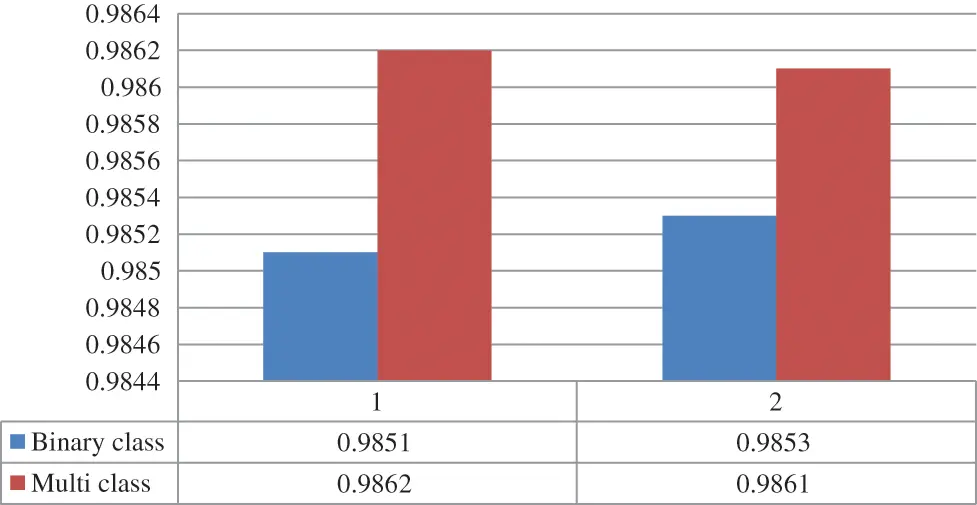Figure 7: Representation of binary and multi-classification

The preprocessing duration is compared to the previous models in Fig. 8. When compared to previous approaches, the suggested model requires 29% less data preprocessing.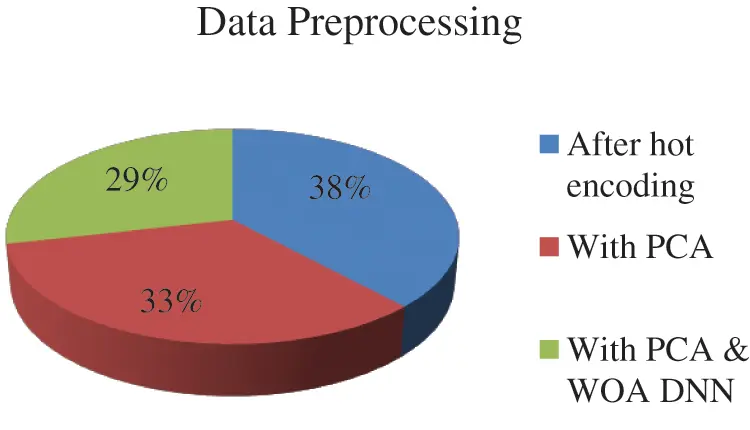Figure 8: Data preprocessing

Fig. 9 compares the proposed model WOA-evaluation DNN’s metrics to those of existing models. When compared to previous models, the suggested model’s evaluation metrics are higher and exhibit an accuracy of 99.1%.WOA-DNN also has a higher attack detection rate compared with others so false alarms are less in WOA-DNN.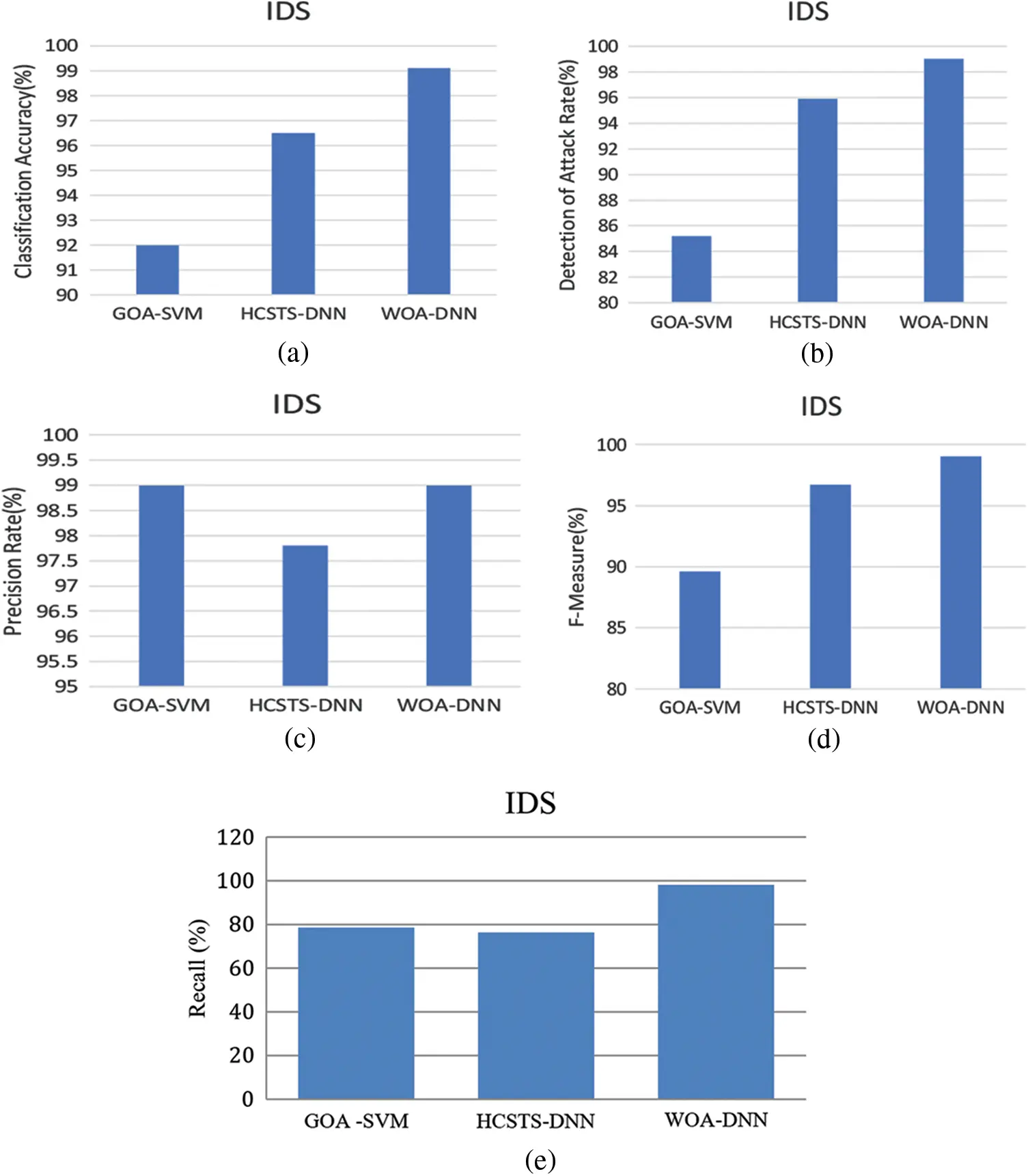Figure 9: Comparison of evaluation metrics (a) classification accuracy (AC) (b) attack rate (AR) (c) precision rate (PR) (d) F- measure (e) Recall

The proposed WOA-DNN is compared with the other models for each of the target classes and normal class. It can be seen from a fig that the WOA-DNN is more accurate in detecting intrusions. Tab. 4 and Figs. 9, 10 shows the accuracy (%) of the proposed model and other methods for the NSL-KDD dataset.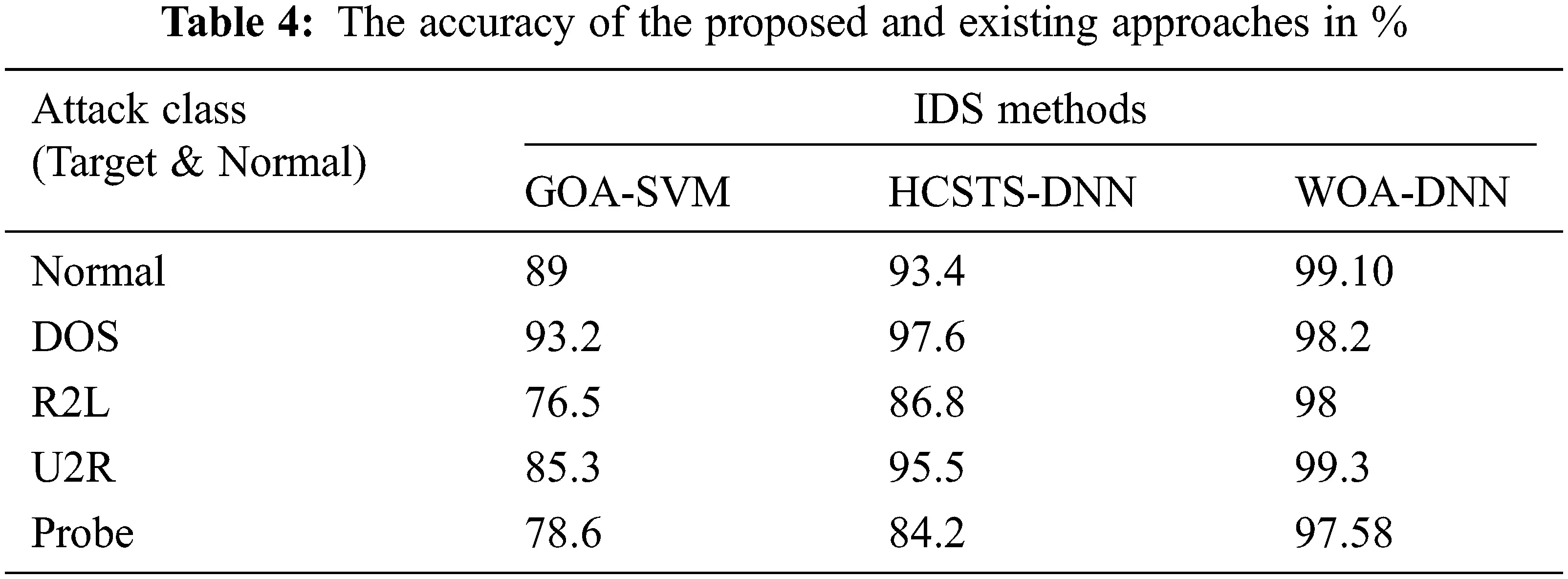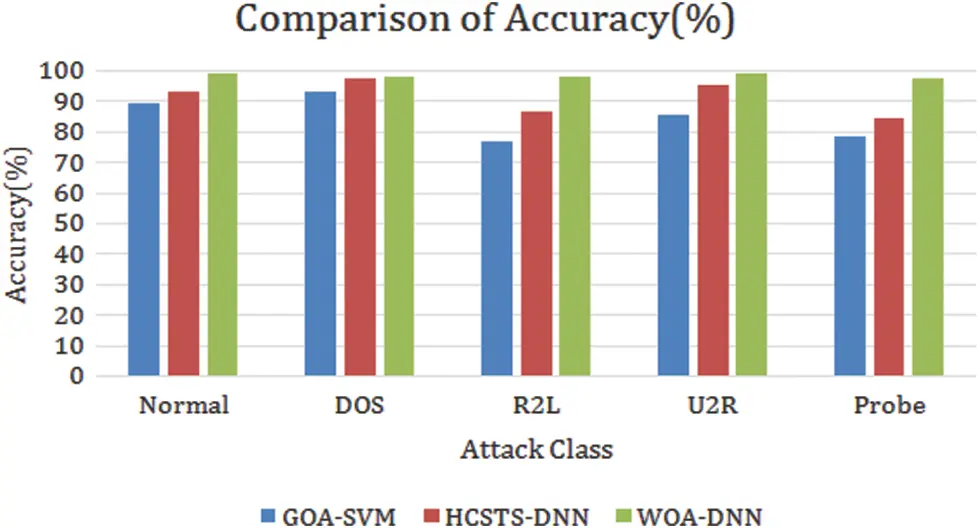Figure 10: The accuracy of the proposed and existing approaches in %

5  Conclusion

This study presents a method for identifying and quantifying intrusions in MANET using WOA-DNN Model. The Proposed method is used to detect intrusions in MANET services. The proposed method reduces the dataset dimensionality using PCA and removes irrelevant data by using the WOA algorithm. This helps in increasing the model’s classification accuracy utilising the DNN Classifier The results show that the metrics utilised to evaluate the proposed method’s effectiveness when contrasted to other approaches are accurate have higher classification accuracy, attack detection rate, and F-Measure. In comparison to existing models, the proposed strategy has a higher attack detection rate of 2.043% than the HCSTS-DNN model and 13.201% than GOA-SVM. The Proposed method has a classification accuracy of 99.1%. The classification accuracy of the proposed WOA-DNN model is 99.1%. The accuracy (%) for the target and normal class is also higher in comparison to other methods. This shows showing the suggested model is quite efficient in detecting MANET intrusions systems. In the future, we’d like to experiment with alternative settings to see if our system can provide a greater attack detection rate with a larger number of assessment metrics and features.

Acknowledgement: The author expressed their heartfelt thanks to the supervisor for his direction and unwavering support that during study.

Funding Statement: The authors received no specific funding for this study.

Conflicts of Interest: The authors declare that they have no conflicts of interest to report regarding the present study.

## References

1. H. Liu and B. Lang, “Machine learning and deep learning methods for intrusion detection systems: A survey,” Applied Sciences, vol. 9, no. 20, pp. 4396, 2019.
2. O. Faker and E. Dogdu, “Intrusion detection using big data and deep learning techniques,” in Proc. ACM Southeast Conf., Kennesaw, GA, USA, pp. 86–93, 2019.
3. P. Wei, Y. Li, Z. Zhang, T. Hu, Z. Li et al., “An optimization method for intrusion detection classification model based on deep belief network,” IEEE Access, vol. 7, pp. 87593–87605, 2019.
4. S. P. Rm, P. K. R. Maddikunta, M. Parimala, S. Koppu, T. R. Gadekallu et al., “An effective feature engineering for DNN using hybrid PCA-GWO for intrusion detection in IoMT architecture,” Computer Communications, vol. 160, no. 6, pp. 139–149, 2020.
5. F. Amato, N. Mazzocca, F. Moscato and E. Vivenzio, “Multilayer perceptron: An intelligent model for classification and intrusion detection,” in Proc. Int. Conf. on Advanced Information Networking and Applications Workshops (WAINA), Taipei, Taiwan, IEEE, pp. 686–691, 2017.
6. S. Uyyala and D. Naik, “Anomaly based intrusion detection of packet dropping attacks in mobile ad-hoc networks,” in Proc. Int. Conf. on Control, Instrumentation, Communication and Computational Technologies (ICCICCT), Kanyakumari, India, IEEE, pp. 1137–1140, 2014.
7. P. J. Chuang and S. H. Li, “Network intrusion detection using hybrid machine learning,” in Proc. Int. Conf. on Fuzzy Theory and Its Applications (iFUZZY), New Taipei, Taiwan, IEEE, pp. 1–5, 2019.
8. K. Amarasinghe and M. Manic, “Improving user trust on deep neural networks-based intrusion detection systems,” in Proc. Annual Conf. of the IEEE Industrial Electronics Society, Washington, DC, USA, pp. 3262–3268, 201
9. M. Maithem and G. A. A. Sultany, “Network intrusion detection system using deep neural networks,” Journal of Physics: Conference Series, vol. 1804, no. 1, pp. 012138, 2021.
10. W. F. Zheng, “Intrusion detection based on convolutional neural network,” in Proc. Int. Conf. on Computer Engineering and Application (ICCEA), Guangzhou, China, pp. 273–277, 2020.
11. M. S. E. Sayed, N. A. L. Khac, M. A. Albahar and A. Jurcut, “A novel hybrid model for intrusion detection systems in SDNs based on CNN and a new regularization technique,” Journal of Network and Computer Applications, vol. 191, no. 4, pp. 103160, 2021.
12. S. Rajabi, S. Jamali and J. Javidan, “An intrusion detection system in computer networks using the firefly algorithm and the fast learning network,” International Journal of Web Research, vol. 3, no. 1, pp. 50–56, 2020.
13. Z. Wang, Y. Liu, D. He and S. Chan, “Intrusion detection methods based on integrated deep learning model,” Computers & Security, vol. 103, no. 6, pp. 102177, 2021.
14. A. Thirumalairaj and M. Jeyakarthic, “Hybrid cuckoo search optimization based tuning scheme for deep neural network for intrusion detection systems in cloud environment,” Journal of Research on the Lepidoptera, vol. 51, no. 2, pp. 209–224, 2020.
15. Z. Ye, Y. Sun, S. Sun, S. Zhan, H. Yu et al., “Research on network intrusion detection based on support vector machine optimized with grasshopper optimization algorithm,” in Proc. IEEE Int. Conf. on Intelligent Data Acquisition and Advanced Computing Systems: Technology and Applications (IDAACS), Metz, France, vol.1, pp. 378–383, 2019.
16. S. Mirjalili and A. Lewis, “The whale optimization algorithm,” Advances in Engineering Software, vol. 95, no. 12, pp. 51–67, 20
17. J. Zhao, Y. Zhao, J. Li, K. Yan and Y. Tian, “Heterogeneous relational complement for vehicle re-identification,” in Proc. of the IEEE/CVF Int. Conf. on Computer Vision, Montreal, QC, Canada, pp. 205–214, 2021.
18. W. Sun, L. Dai, X. R. Zhang, P. S. Chang and X. Z. He, “RSOD: Real-time Small object detection algorithm in UAV-based traffic monitoring,” Applied Intelligence, vol. 92, no. 6, pp. 1–16, 2021.This work is licensed under a Creative Commons Attribution 4.0 International License, which permits unrestricted use, distribution, and reproduction in any medium, provided the original work is properly cited.